"Web Equation" which recognizes handwritten mathematical expressions automatically to LaTeX or MathML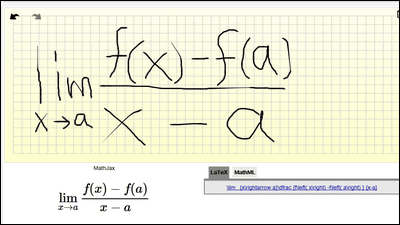If you write expressions in the specified area with a mouse, it will automatically recognize and analyze in about 1 second or so,LaTeXYaMathMLIt converts it and outputs a free service for this "Web Equation"is.

Web Equation
https://webdemo.myscript.com/#/demo/equation

This is the initial screen, if you write a formula here with a mouse it will automatically recognize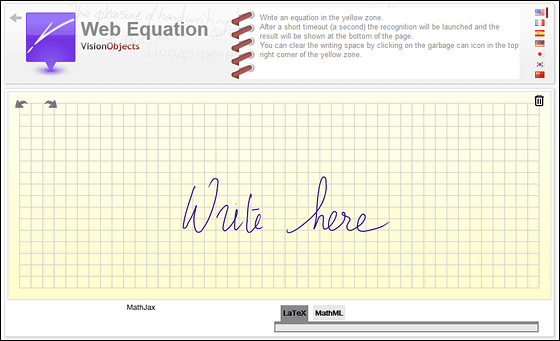Choosing Japan from the upper right flag will look like this. I wrote it because I was told "write here".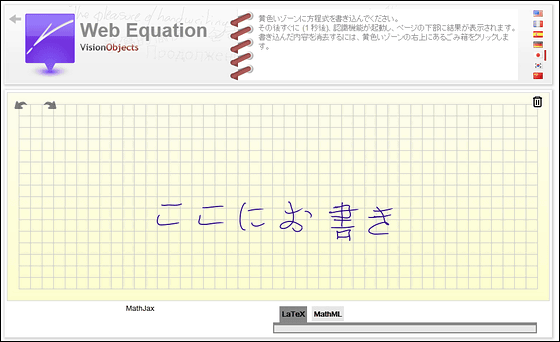Such complex expressions are also OK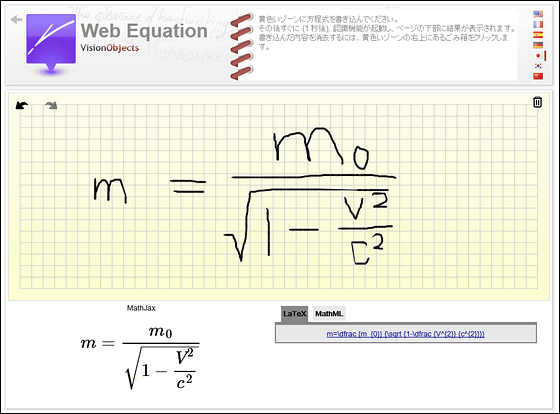If you click the tab of "MathML" it will be output like this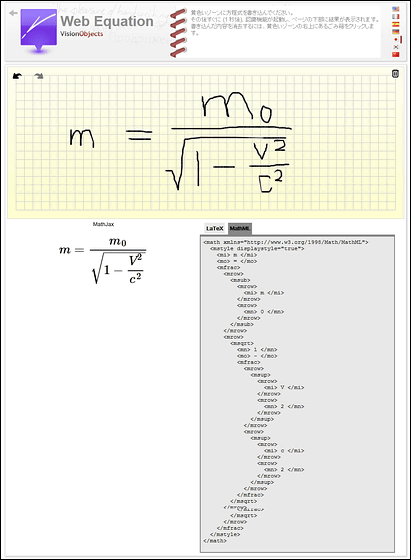Patterns will recognize even if it is somewhat dirty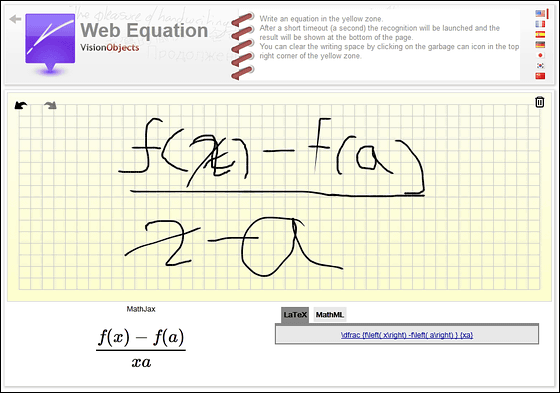I could recognize the arrow, it is quite convenient and excellent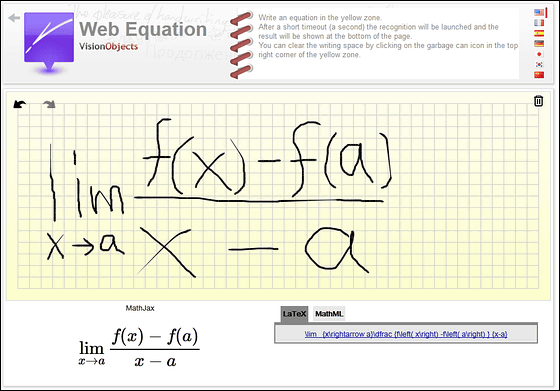in Review,   Web Service, Posted by darkhorse